• +91 9971497814
• info@interviewmaterial.com

# Chapter 10- Mechanical Properties Of Fluids Interview Questions Answers

### Related Subjects

Question 1 :
Explain why
(a) The blood pressure in humans is greater at the feet than at the brain
(b) Atmospheric pressure at a height of about 6 km decreases to nearly half of its value at the sea level, though the height of the atmosphere is more than 100 km
(c) Hydrostatic pressure is a scalar quantity even though pressure is force divided by area.

(a) The pressure of a liquid is given by the relation:
P = hρg
Where,
P = Pressure
h = Height of the liquid column
ρ = Density of the liquid
g = Acceleration due to the gravity
It can be inferred that pressure is directly proportional to height. Hence, the blood pressure in human vessels depends on the height of the blood column in the body. The height of the blood column is more at the feet than it is at the brain. Hence, the blood pressure at the feet is more than it is at the brain.
(b) Density of air is the maximum near the sea level. Density of air decreases with increase in height from the surface. At a height of about 6 km, density decreases to nearly half of its value at the sea level. Atmospheric pressure is proportional to density. Hence, at a height of 6 km from the surface, it decreases to nearly half of its value at the sea level.
(c) When force is applied on a liquid, the pressure in the liquid is transmitted in all directions. Hence, hydrostatic pressure does not have a fixed direction and it is a scalar physical quantity.

Question 2 :
Explain why
(a) The angle of contact of mercury with glass is obtuse, while that of water with glass is acute.
(b) Water on a clean glass surface tends to spread out while mercury on the same surface tends to form drops. (Put differently, water wets glass while mercury does not.)
(c) Surface tension of a liquid is independent of the area of the surface
(d) Water with detergent dissolved in it should have small angles of contact.
(e) A drop of liquid under no external forces is always spherical in shape

Answer 2 : (a) The angle between the tangent to the liquid surface at the point of contact and the surface inside the liquid is called the angle of contact (θ), as shown in the given figure.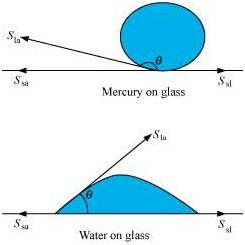SlaSsa, and Ssl are the respectiveinterfacial tensions between the liquid-air, solid-air, and solid-liquidinterfaces. At the line of contact, the surface forces between the three mediamust be in equilibrium, i.e.,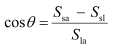The angleof contact θ , is obtuse if Ssa < Sla (as in the case ofmercury on glass). This angle is acute if Ssl la (as inthe case of water on glass).

(b) Mercury molecules (which make an obtuse angle with glass) have a strong force of attraction between themselves and a weak force of attraction toward solids. Hence, they tend to form drops.

On the other hand, water molecules make acute angles with glass. They have a weak force of attraction between themselves and a strong force of attraction toward solids. Hence, they tend to spread out.

(c) Surface tension is the force acting per unit length at the interface between the plane of a liquid and any other surface. This force is independent of the area of the liquid surface. Hence, surface tension is also independent of the area of the liquid surface.

(d) Water with detergent dissolved in it has small angles of contact (θ). This is because for a small θ, there is a fast capillary rise of the detergent in the cloth. The capillary rise of a liquid is directly proportional to the cosine of the angle of contact (θ). If θ is small, then cosθ will be large and the rise of the detergent water in the cloth will be fast.

(e) A liquid tends to acquire the minimum surface area because of the presence of surface tension. The surface area of a sphere is the minimum for a given volume. Hence, under no external forces, liquid drops always take spherical shape.

Question 3 :
Fill in the blanks using the word(s) from the list appended with each statement:
(a) Surface tension of liquids generally . . . with temperatures (increases / decreases)
(b) Viscosity of gases. .. with temperature, whereas viscosity of liquids . . . with temperature (increases / decreases)
(c) For solids with elastic modulus of rigidity, the shearing force is proportional to . . . , while for fluids it is proportional to . .. (shear strain / rate of shear strain)
(d) For a fluid in a steady flow, the increase in flow speed at a constriction follows (conservation of mass / Bernoulli’s principle)
(e) For the model of a plane in a wind tunnel, turbulence occurs at a ... speed for turbulence for an actual plane (greater / smaller)

(a) decreases
The surface tension of a liquid is inversely proportional to temperature.
(b) increases; decreases
Most fluids offer resistance to their motion. This is like internal mechanical friction, known as viscosity. Viscosity of gases increases with temperature, while viscosity of liquids decreases with temperature.
(c) Shear strain; Rate of shear strain
With reference to the elastic modulus of rigidity for solids, the shearing force is proportional to the shear strain. With reference to the elastic modulus of rigidity for fluids, the shearing force is proportional to the rate of shear strain.
(d) Conservation of mass/Bernoulli’s principle
For a steady-flowing fluid, an increase in its flow speed at a constriction follows the conservation of mass/Bernoulli’s principle.
(e) Greater
For the model of a plane in a wind tunnel, turbulence occurs at a greater speed than it does for an actual plane. This follows from Bernoulli’s principle and different Reynolds’ numbers are associated with the motions of the two planes.

Question 4 :
Explain why
(a) To keep a piece of paper horizontal, you should blow over, not under, it
(b) When we try to close a water tap with our fingers, fast jets of water gush through the openings between our fingers
(c) The size of the needle of a syringe controls flow rate better than the thumb pressure exerted by a doctor while administering an injection
(d) A fluid flowing out of a small hole in a vessel results in a backward thrust on the vessel
(e) A spinning cricket ball in air does not follow a parabolic trajectory

(a) When air is blown under a paper, the velocity of air is greater under the paper than it is above it. As per Bernoulli’s principle, atmospheric pressure reduces under the paper. This makes the paper fall. To keep a piece of paper horizontal, one should blow over it. This increases the velocity of air above the paper. As per Bernoulli’s principle, atmospheric pressure reduces above the paper and the paper remains horizontal.
(b) According to the equation of continuity:
Area × Velocity = Constant
For a smaller opening, the velocity of flow of a fluid is greater than it is when the opening is bigger. When we try to close a tap of water with our fingers, fast jets of water gush through the openings between our fingers. This is because very small openings are left for the water to flow out of the pipe. Hence, area and velocity are inversely proportional to each other.
(c) The small opening of a syringe needle controls the velocity of the blood flowing out. This is because of the equation of continuity. At the constriction point of the syringe system, the flow rate suddenly increases to a high value for a constant thumb pressure applied.
(d) When a fluid flows out from a small hole in a vessel, the vessel receives a backward thrust. A fluid flowing out from a small hole has a large velocity according to the equation of continuity:
Area × Velocity = Constant
According to the law of conservation of momentum, the vessel attains a backward velocity because there are no external forces acting on the system.
(e) A spinning cricket ball has two simultaneous motions – rotatory and linear. These two types of motion oppose the effect of each other. This decreases the velocity of air flowing below the ball. Hence, the pressure on the upper side of the ball becomes lesser than that on the lower side. An upward force acts upon the ball. Therefore, the ball takes a curved path. It does not follow a parabolic path.

Question 5 : A 50 kg girl wearing high heel shoes balances on a single heel. The heel is circular with a diameter 1.0 cm. What is the pressure exerted by the heel on the horizontal floor?

Mass of the girl, m = 50 kg

Diameterof the heel, d = 1 cm = 0.01 m

Radius ofthe heel, r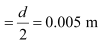Area ofthe heel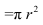= π (0.005)2

= 7.85 ×10–5 m2

Forceexerted by the heel on the floor:

F = mg

= 50 ×9.8

= 490 N

Pressureexerted by the heel on the floor: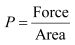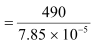= 6.24 ×106 N m–2

Therefore,the pressure exerted by the heel on the horizontal floor is
6.24 × 106 Nm–2.

Question 6 : Toricelli’s barometer used mercury. Pascal duplicated it using French wine of density 984 kg m–3. Determine the height of the wine column for normal atmospheric pressure.

10.5 m

Density of mercury, ρ1 = 13.6 × 103 kg/m3

Height of the mercury column, h1 =0.76 m

Density of French wine, ρ2 = 984 kg/m3

Height of the French wine column = h2

Acceleration due to gravity, g = 9.8 m/s2

The pressure in both the columns is equal, i.e.,

Pressure in the mercury column = Pressure in the French winecolumn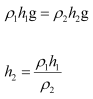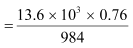= 10.5 m

Hence, the height of the French wine column for normal atmosphericpressure is 10.5 m.

Question 7 : A vertical off-shore structure is built to withstand a maximum stress of 109 Pa. Is the structure suitable for putting up on top of an oil well in the ocean? Take the depth of the ocean to be roughly 3 km, and ignore ocean currents.

Yes

The maximum allowable stress for the structure, P =109 Pa

Depth of the ocean, d = 3 km = 3 × 103 m

Density of water, ρ = 103 kg/m3

Acceleration due to gravity, g = 9.8 m/s2

The pressure exerted because of the sea water at depth, ρdg

= 3 × 103 × 103 × 9.8

= 2.94 × 107 Pa

Themaximum allowable stress for the structure (109 Pa) is greater thanthe pressure of the sea water (2.94 × 107 Pa). The pressureexerted by the ocean is less than the pressure that the structure canwithstand. Hence, the structure is suitable for putting up on top of an oilwell in the ocean

Question 8 : A hydraulic automobile lift is designed to lift cars with a maximum mass of 3000 kg. The area of cross-section of the piston carrying the load is 425 cm2. .What maximum pressure would the smaller piston have to bear?

The maximum mass of a car that can be lifted, m =3000 kg

Area of cross-section of the load-carrying piston, A =425 cm= 425 × 10–4 m2

The maximum force exerted by the load, F = mg

= 3000 × 9.8

= 29400 N

The maximum pressure exerted on the load-carrying piston,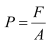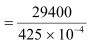= 6.917 × 105 Pa

Pressure is transmitted equally in all directions in a liquid.Therefore, the maximum pressure that the smaller piston would have to bear is6.917 × 105 Pa.

Question 9 : A U-tube contains water and methylated spirit separated by mercury. The mercury columns in the two arms are in level with 10.0 cm of water in one arm and 12.5 cm of spirit in the other. What is the specific gravity of spirit?

The given system of water, mercury, and methylated spirit is shownas follows: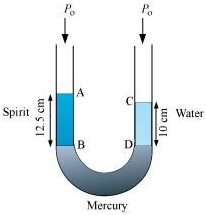Height of the spirit column, h1 = 12.5cm = 0.125 m

Height of the water column, h2 = 10 cm= 0.1 m

P0 =Atmospheric pressure

ρ1 =Density of spirit

ρ2 =Density of water

Pressure at point B =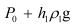Pressure at point D =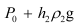Pressure at points B and D is the same.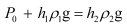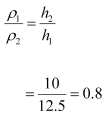Therefore, the specific gravity of spirit is 0.8.

Question 10 : In problem 10.9, if 15.0 cm of water and spirit each are further poured into the respective arms of the tube, what is the difference in the levels of mercury in the two arms? (Specific gravity of mercury = 13.6)

Height of the water column, h1 = 10 +15 = 25 cm

Height of the spirit column, h2 = 12.5+ 15 = 27.5 cm

Density of water, ρ1 = 1 g cm–3

Density of spirit, ρ2 = 0.8 g cm–3

Density of mercury = 13.6 g cm–3

Let h be the difference between the levels ofmercury in the two arms.

Pressure exerted by height h, of the mercury column:

g

h × 13.6g … (i)

Difference between the pressures exerted by water and spirit: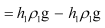= g(25 × 1 – 27.5 × 0.8)

= 3g … (ii)

Equating equations (i) and (ii), we get:

13.6 hg = 3g

h = 0.220588 ≈ 0.221 cm

Hence, the difference between the levels of mercury in the twoarms is 0.221 cm.

Todays Deals### Chapter 10- Mechanical Properties Of Fluids Contributorskrishan

Name:
Email:

# Latest News# 9000 interview questions in different categories